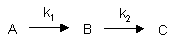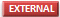# B2. Multi-Step Reactions

## Reversible First Order Reactions

$A \underset{k_2} {\overset{k_1}{\rightleftharpoons}} P$

A differential equation can be written for this reaction:

$v = \dfrac{d[A]}{dt} = -k_1[A] + k_2[P] \label{7}$

This can be solved through integration to give the following equations:Graphs of A and P vs t for this reaction at two different sets of values of k1 and k2 are shown below.

Figure: Reversible First Order Reactions: A <=> PXcel Spread Sheet: Reversible First Order Reactions -

Go to the following spread sheet and change the values of k1 and k2. Note the changes in the graphs. Remember from our discussion of macromolecule:ligand binding, the dissociation constant, Kd, was related to the rate constants by the formula Kd = k2/k1. Note that if the first order rate constants for a reversible chemical reaction are equal, Keq (and its inverse) equal 1, and the equilibrium concentrations of A and P are equal.4/26/13Wolfram Mathematica CDF Player - Reversible First Order Reactions ([A] blue, [B] red) (free plugin required)

## Consecutive First Order ReactionsFor these reactions:Graphs of A, B, and C vs t for these reaction at two different sets of values of k1 and k2 are shown below.Figure: Consecutive Irreversible First Order Reactions: A --> B --> CXcel Spread Sheet: Consecutive Reactions -

Change the values of k1 and k2. Note the changes in the graphs.4/26/13Wolfram Mathematica CDF Player - Irreversible Consecutive First Order Reactions ([A] blue, [B] red, [C] orange (free plugin required)Reaction Appliets:

•Reactions Kinetics: Java Applet - Zero, First, and Second Order Reactions
•Consecutive reactions
•Graphical determination of reaction order from initial rates